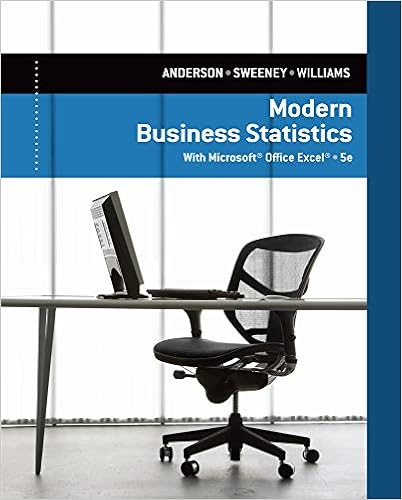# You own a portfolio equally invested in a risk free

• Test Prep
• 81
• 91% (149) 135 out of 149 people found this document helpful

This preview shows page 79 - 81 out of 81 pages.

##### We have textbook solutions for you!
The document you are viewing contains questions related to this textbook.The document you are viewing contains questions related to this textbook.
Chapter 3 / Exercise 60
Modern Business Statistics with Microsoft Excel
Anderson/SweeneyExpert Verified
94. You own a portfolio equally invested in a risk-free asset and two stocks. If one of the stocks has a beta of 1.86 and the total portfolio is equally as risky as the market, what must the beta be for the otherstock in your portfolio?A. 1.07B. .54C.1.14D. .14E. .97P = 1 = (1/3)(0) + (1/3)(1.86) + (1/3)(x)x = 1.14AACSB: Analytical ThinkingAccessibility: Keyboard NavigationBlooms: AnalyzeDifficulty: 1 BasicLearning Objective: 11-03 Define the systematic risk principle.Section: 11.7 The Security Market LineTopic: Beta95. A stock has a beta of 1.32, the expected return on the market is 12.72, and the risk-free rate is 4.05. What must the expected return on this stock be?A. 16.67 percentB. 14.75 percentC. 17.10 percentD. 20.46 percentE.15.49 percentE(R) = .0405 + 1.32(.1272 -.0405) = .1549, or 15.49 percentAACSB: Analytical ThinkingAccessibility: Keyboard NavigationBlooms: AnalyzeDifficulty: 1 BasicLearning Objective: 11-04 Discuss the security market line and the risk-return trade-off.Section: 11.7 The Security Market LineTopic: Capital asset pricing model96. A stock has an expected return of 14.3 percent, the risk-free rate is 3.9 percent, and the market riskpremium is 7.8 percent. What must the beta of this stock be?A. 1.67B. .94C. 1.08D. 1.21E.1.33E(R) = .143 = .039 + (.078) = 1.33 AACSB: Analytical ThinkingAccessibility: Keyboard NavigationBlooms: AnalyzeDifficulty: 1 BasicLearning Objective: 11-04 Discuss the security market line and the risk-return trade-off.Section: 11.7 The Security Market LineTopic: Capital asset pricing model
##### We have textbook solutions for you!
The document you are viewing contains questions related to this textbook.The document you are viewing contains questions related to this textbook.
Chapter 3 / Exercise 60
Modern Business Statistics with Microsoft Excel
Anderson/SweeneyExpert Verified
97. A stock has a beta of 1.48 and an expected return of 17.3 percent. A risk-free asset currently earns 4.6 percent. If a portfolio of the two assets has a beta of .98, then the weight of the stock must be ___ and the risk-free weight must be___.A. .56; .44B. .34; .66C. .44; .56D..66; .34E. .72; .28P = .98 = x(1.48) + (1 –x)(0)x = .66Stock weight is .66 and the risk-free weight is .34AACSB: Analytical ThinkingAccessibility: Keyboard NavigationBlooms: AnalyzeDifficulty: 1 BasicLearning Objective: 11-04 Discuss the security market line and the risk-return trade-off.Section: 11.6 Systematic Risk and BetaTopic: Beta98. Stock Y has a beta of 1.28 and an expected return of 13.7 percent. Stock Z has a beta of 1.02 and an expected return of 11.4 percent. What would the risk-free rate have to be for the two stocks to be correctly priced relative to each other?A.2.38 percentB. 2.76 percentC. 3.23 percentD. 3.69 percentE. 4.08 percent(.137 -Rf)/1.28 = (.114 -Rf)/1.02 Rf = .0238, or 2.38 percentAACSB: Analytical ThinkingAccessibility: Keyboard NavigationBlooms: AnalyzeDifficulty: 1 BasicLearning Objective: 11-04 Discuss the security market line and the risk-return trade-off.Section: 11.7 The Security Market LineTopic: Reward-to-risk ratio
•••